# Flag structure

(diff) ← Older revision | Latest revision (diff) | Newer revision → (diff)

The same as a flag.

A flag structure of type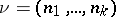on an-dimensional manifoldis a field of flagsof typedefined by subspaces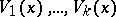of the tangent spaces, depending smoothly on the point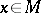. In other words, a flag structure of typeonis a smooth section of the bundle of flags of typeon, the typical fibre of which at the pointis the variety. A flag structure of type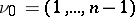is called complete or full. A flag structure of typeon a manifold is a-structure, whereis the group of all linear transformations of the-dimensional vector space preserving some flag of type. This-structure is of infinite type. The automorphism group of a flag structure is, generally speaking, infinite-dimensional. The Lie algebraof infinitesimal automorphisms of a flag structure onhas a chain of ideals, where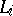consists of the vector fields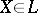such that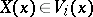for all.

An important special case of flag structures are those of type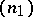, or-dimensional distributions (here,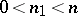).

A flag structure of typeonis called locally flat, or integrable, if every point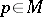has a neighbourhoodand a coordinate system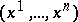in it such that the subspaceis spanned by the vectorsfor all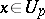and all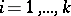. This means that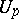has a collection of foliationssuch that for allthe flag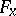is defined by a collection of subspaces of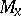tangent to the leaves of these foliations passing through. A flag structure is locally flat if and only if for everythe distributionis involutory, that is, if for any two vector fieldsandonsuch thatand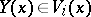for all, it is true that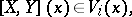where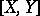is the Lie bracket ofand.

The existence of global (everywhere-defined) flag structures on a manifold imposes fairly-strong restrictions on its topological structure. For example, there is a line field, that is, a flag structure of type , on a simply-connected compact manifold if and only if its Euler characteristic vanishes. There is a complete flag structure on a simply-connected manifold if and only if it is completely parallelizable, that is, if its tangent bundle is trivial. If there is a parallel flag structure of type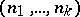that is invariant relative to parallel displacements on a complete simply-connected-dimensional Riemannian manifold, thenis isomorphic to the direct product of simply-connected Riemannian manifolds of dimensions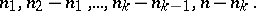A transitive group of diffeomorphisms of a manifoldleaves some flag structure of typeoninvariant if and only if its linear isotropy group preserves some flag of typein the tangent space to. In particular, ifis a closed subgroup of a Lie groupsuch that the restriction toof the adjoint representation ofgives a triangular linear group, then there is an invariant complete flag structure on the homogeneous space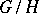, and also an invariant flag structure of every other type.

A theory of deformations of flag structures on compact manifolds has been developed .

How to Cite This Entry:
Flag structure. Encyclopedia of Mathematics. URL: http://encyclopediaofmath.org/index.php?title=Flag_structure&oldid=17937
This article was adapted from an original article by D.V. Alekseevskii (originator), which appeared in Encyclopedia of Mathematics - ISBN 1402006098. See original article